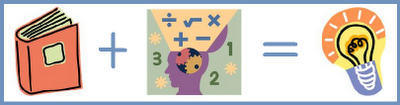## Monday, July 30, 2012

### Reading-Research Monday: Reading + Math = Learning

Reading and Math.  Many assume that these two subjects are polar opposites—that someone is either a reading person or math minded, but that doesn’t need to be the case, and teachers’ instructional strategies shouldn’t be limited by this assumption.Reading + Math = Learning

In the paper “A Review and an Update on Using Children’s Literature to Teach Mathematics” published in 2008, author June Gatsón discusses how literature can be used to teach math.  In her review, Gatsón found that when teachers use literature as a connection to and a resource for their mathematics instruction, students become more interested and motivated, develop better critical thinking and problem solving skills, are able to see connections between math and their lives, and are able to recognize that math is a life-long tool.

Gatsón cites Welchman-Tischler (1992) when she lists seven ways to implement literature into mathematics lessons:
1. To provide a context or model for an activity with mathematical content
2. To introduce manipulatives that will be used in varied ways (not necessarily as in the story)
3. To inspire a creative mathematics experience for children
4. To pose an interesting problem
5. To prepare for a mathematics concept or skill
6. To develop or explain a mathematics concept or skill
7. To review a mathematics concept or skill
While this list does provide a foundation for how to implement a math-reading instructional strategy, it still remains somewhat abstract. To give more concrete and reproducible ideas, Gatsón provides links to the following websites as resources for teachers interested in using reading as an instructional tool when teaching math:

 Website Description Comprehensive list of books divided by category (fractions, add & subtract, Geometry, etc.) and by grade level Three lessons that illustrate how literature and mathematics instruction can be connected Broad list of lesson plans broken down by grade level or math strand Story mapping technology to create math stories

The books teachers use do not have to be math concept books or even directly related to mathematics.  These types of books obviously can be very helpful when relating math and reading since the content of the book clearly links the two together.  However, any book can be used.  Is there a book all students are reading?  A book they all love?  A book they can’t stop talking about.  Teachers can use these popular stories as a background for mathematics instruction.  For example, it would be easy to make a mathematical story problems based on important events in the book.

For additional strategies and for lists of resources, five more websites are listed below.

 Website Description A PowerPoint presentation in a pdf file that demonstrates how to use reading and writing strategies to solve math problems Discusses effective math instruction using children’s literature in a Q&A format A sample lesson plan from scholastic Provides an overview and advice for starting literature-based mathematics lessons Books listed by math strand with ideas for instruction listed next to each book

Math and reading do not need to be separated.  And in fact, when used in combination with one another, positive results are seen in student achievement.

What do you think?  Do you or have you used reading in your math lessons?  Would you ever try it?

#### Post a Comment